Updating search results...

# 195 Results

View
Selected filters:
• Geometry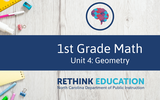Conditional Remix & Share Permitted
CC BY-NC-SA
Rating
0.0 stars
Overview:

This unit was created by the Rethink Education Content Development Team. This course is aligned to the NC Standards for 1st Grade Math for Geometry.

Subject:
Geometry
Material Type:
Activity/Lab
Assessment
Formative Assessment
Homework/Assignment
Presentation
Unit of Study
Vocabulary
Author:
AMBER GARVEY
02/15/2023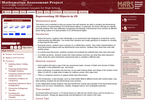Only Sharing Permitted
CC BY-NC-ND
Rating
4.5 stars
Overview:

This lesson unit is intended to help teachers assess how well students are able to visualize two-dimensional cross-sections of representations of three-dimensional objects. In particular, the lesson will help you identify and help students who have difficulties recognizing and drawing two-dimensional cross-sections at different points along a plane of a representation of a three-dimensional object.

Subject:
Geometry
Material Type:
Assessment
Lesson Plan
Provider:
Shell Center for Mathematical Education
Provider Set:
Mathematics Assessment Project (MAP)
06/24/2019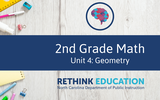Conditional Remix & Share Permitted
CC BY-NC-SA
Rating
0.0 stars
Overview:

This unit was created by the Rethink Education Content Development Team. This course is aligned to the NC Standards for 2nd Grade Math in Geometry.

Subject:
Geometry
Material Type:
Activity/Lab
Assessment
Formative Assessment
Homework/Assignment
Presentation
Unit of Study
Vocabulary
Author:
AMBER GARVEY
02/17/2023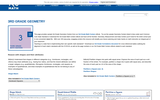Rating
4.0 stars
Overview:

Great for math centers that align with the 3rd grade Geometry unit!

Subject:
Geometry
Material Type:
Activity/Lab
Author:
© 2010 - 2019 K-5 Math Teaching Resources LLC
07/29/2019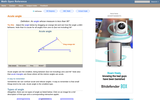Rating
5.0 stars
Overview:

An interactive applet and associated web page that demonstrate acute angles (those less than 90 deg). The applet presents an angle (initially acute) that the user can adjust by dragging the end points of the line segments forming the angle. As it changes it shows the angle measure and a message that indicate which type of angle it is. There a software 'detents' that make it easy capture exact angles such as 90 degrees and 180 degrees The message and angle measures can be turned off to facilitate classroom discussion. The text on the page has links to other pages defining each angle type in depth. Applet can be enlarged to full screen size for use with a classroom projector. This resource is a component of the Math Open Reference Interactive Geometry textbook project at http://www.mathopenref.com.

Subject:
Geometry
Material Type:
Simulation
Provider:
Math Open Reference
Author:
John Page
05/07/2019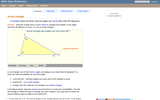Rating
0.0 stars
Overview:

An interactive applet and associated web page that demonstrate the three types of triangle: acute, obtuse and right. The applet shows a triangle that is initially acute (all angles less then 90 degrees) which the user can reshape by dragging any vertex. There is a message changes in real time while the triangle is being dragged that tells if the triangle is an acute, right or obtuse triangle and gives the reason why. By experimenting with the triangle student can develop an intuitive sense of the difference between these three classes of triangle. Applet can be enlarged to full screen size for use with a classroom projector. This resource is a component of the Math Open Reference Interactive Geometry textbook project at http://www.mathopenref.com.

Subject:
Geometry
Material Type:
Simulation
Provider:
Math Open Reference
Author:
John Page
05/07/2019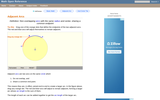Rating
0.0 stars
Overview:

An interactive applet and associated web page that demonstrate the the concept of adjacent arcs. The user can 3 drag points around a circle that define the endpoints of two adjacent arcs. Web page has the properties of adjacent arcs. Applet can be enlarged to full screen size for use with a classroom projector. This resource is a component of the Math Open Reference Interactive Geometry textbook project at http://www.mathopenref.com.

Subject:
Geometry
Material Type:
Simulation
Provider:
Math Open Reference
Author:
John Page
05/07/2019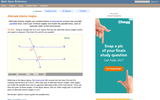Rating
0.0 stars
Overview:

An interactive applet and associated web page that demonstrate the alternate interior angles that are formed where a transversal crosses two lines. The applets shows the two possible pairs of angles alternating when in animation mode. By dragging the three lines, it can be seen that the angles are congruent only when the lines are parallel. When not in animated mode, there is a button that alternates the two pairs of angles. The text on the page discusses the properties of the angle pairs both in the parallel and non-parallel cases. Applet can be enlarged to full screen size for use with a classroom projector. This resource is a component of the Math Open Reference Interactive Geometry textbook project at http://www.mathopenref.com.

Subject:
Geometry
Material Type:
Simulation
Provider:
Math Open Reference
Author:
John Page
05/07/2019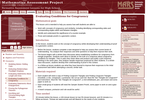Only Sharing Permitted
CC BY-NC-ND
Rating
5.0 stars
Overview:

This lesson unit is intended to help teachers assess how well students are able to: work with concepts of congruency and similarity, including identifying corresponding sides and corresponding angles within and between triangles; Identify and understand the significance of a counter-example; Prove, and evaluate proofs in a geometric context.

Subject:
Geometry
Material Type:
Assessment
Lesson Plan
Provider:
Shell Center for Mathematical Education
Provider Set:
Mathematics Assessment Project (MAP)
06/24/2019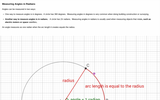Unrestricted Use
CC BY
Rating
3.0 stars
Overview:

The students will be able to define the radian measure of an angle.

Subject:
Geometry
Material Type:
Interactive
Provider:
Michigan Virtual
07/19/2019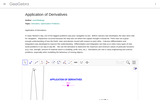Conditional Remix & Share Permitted
CC BY-SA
Rating
0.0 stars
Overview:

We use the derivative to determine the maximum and minimum values of particular functions (e.g. cost, strength, amount of material used in a building, profit, loss, etc.).Differentiation is also used in analysis of finance and economics.

Subject:
Mathematics
Geometry
Material Type:
Simulation
Provider:
GeoGebra
Provider Set:
GeoGebraTube
03/12/2012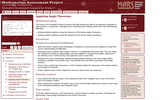Only Sharing Permitted
CC BY-NC-ND
Rating
4.0 stars
Overview:

This lesson unit is intended to help you assess how well students are able to use geometric properties to solve problems. In particular, it will support you in identifying and helping students who have the following difficulties: Solving problems relating to using the measures of the interior angles of polygons; and solving problems relating to using the measures of the exterior angles of polygons.

Subject:
Geometry
Material Type:
Assessment
Lesson Plan
Provider:
Shell Center for Mathematical Education
Provider Set:
Mathematics Assessment Project (MAP)
06/24/2019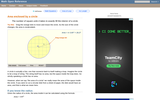Rating
0.0 stars
Overview:

An interactive applet and associated web page that demonstrate the area of a circle. A circle is shown with a point on the circumference that can be dragged to resize the circle. As the circle is resized, the radius and the area computation is shown changing in real time. The radius and formula can be hidden for class discussion. Applet can be enlarged to full screen size for use with a classroom projector. This resource is a component of the Math Open Reference Interactive Geometry textbook project at http://www.mathopenref.com.

Subject:
Geometry
Material Type:
Simulation
Provider:
Math Open Reference
Author:
John Page
05/07/2019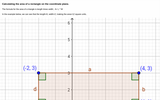Unrestricted Use
CC BY
Rating
2.0 stars
Overview:

Images of rectangle on coordinate plane, one with easily identifiable area, other image with same rectangle rotated. Distance formula shown to calculate same area.

Subject:
Geometry
Material Type:
Interactive
Provider:
Michigan Virtual
07/19/2019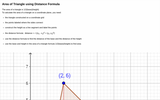Unrestricted Use
CC BY
Rating
1.66666666667 stars
Overview:

Definition of area of triangle, image of triangle on coordinate grid with height constructed. Area of triangle calculated using distance formula.

Subject:
Geometry
Material Type:
Interactive
Provider:
Michigan Virtual
07/19/2019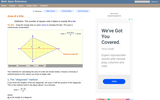Rating
5.0 stars
Overview:

An interactive applet and associated web page that deals with the area of a kite, (a quadrilateral with two distinct pairs of equal adjacent sides). The applet shows a kite and the user can reshape it by dragging any vertex. The other vertices move automatically to ensure it always remains a kite. As the vertices are dragged, the area is continuously recalculated and displayed. The kite is filled with a grid of unit squares so that the students can estimate the area. The on-screen calculation can be hidden until the estimates are done. The web page lists two different ways to compute the area of a kite. Applet can be enlarged to full screen size for use with a classroom projector. This resource is a component of the Math Open Reference Interactive Geometry textbook project at http://www.mathopenref.com.

Subject:
Geometry
Material Type:
Simulation
Provider:
Math Open Reference
Author:
John Page
05/07/2019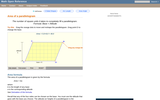Rating
0.0 stars
Overview:

A web page and interactive applet showing the ways to calculate the area of a parallelogram. The user can drag the vertices of the parallelogram and the other points change automatically to ensure it remains a parallelogram. A grid inside the shape allows students to estimate the area visually, then check against the actual computed area, which is continuously recomputed and displayed. The text on the page gives three different ways to calculate the area with a formula for each. The applet uses one of the methods to compute the area in real time, so it changes as the rhombus is reshaped with the mouse. A companion page is http://www.mathopenref.com/parallelogram.html showing the definition and properties of a parallelogram Applet can be enlarged to full screen size for use with a classroom projector. This resource is a component of the Math Open Reference Interactive Geometry textbook project at http://www.mathopenref.com.

Subject:
Geometry
Material Type:
Simulation
Provider:
Math Open Reference
Author:
John Page
05/07/2019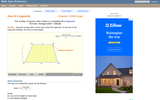Rating
0.0 stars
Overview:

A web page and interactive applet showing the ways to calculate the area of a trapezoid. The user can drag the vertices of the trapezoid and the other points change automatically to ensure it remains a trapezoid. A grid inside the shape allows students to estimate the area visually, then check against the actual computed area. The text on the page gives three different ways to calculate the area with a formula for each. The applet uses one of the methods to compute the area in real time, so it changes as the trapezoid is reshaped with the mouse. A companion page is http://www.mathopenref.com/trapezoid.html showing the definition and properties of a trapezoid. Applet can be enlarged to full screen size for use with a classroom projector. This resource is a component of the Math Open Reference Interactive Geometry textbook project at http://www.mathopenref.com.

Subject:
Geometry
Material Type:
Simulation
Provider:
Math Open Reference
Author:
John Page
05/07/2019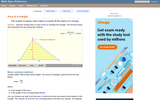Rating
5.0 stars
Overview:

An interactive applet and associated web page that explain the area of a triangle. The applet shows a triangle that can be reshaped by dragging any vertex. As it changes, the area is continually recalculated using the 'half base times height' method. The triangle has a fixed square grid in its interior that can be used to visually estimate the area for later correlation with the calculated value. The calculation can be hidden while estimation is in progress. The text page has links to a similar page that uses Heron's Formula to compute the area. Applet can be enlarged to full screen size for use with a classroom projector. This resource is a component of the Math Open Reference Interactive Geometry textbook project at http://www.mathopenref.com.

Subject:
Geometry
Material Type:
Simulation
Provider:
Math Open Reference
Author:
John Page
05/07/2019Conditional Remix & Share Permitted
CC BY-NC-SA
Rating
0.0 stars
Overview:

This task explores the real world topic of building light rails. Throughout the implementation of this task the students will learn about the cost of building railways and how to implement them within a budget. This task explores such mathematical concepts of using coordinates to find the distance between points, using coordinates to build polygons and find the area and length of sides, and writing equations of parallel lines.

Subject:
Algebra
Geometry
Material Type:
Activity/Lab
Author:
Carrie Robledo
James O'Neal
03/26/2021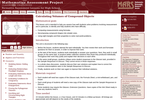Only Sharing Permitted
CC BY-NC-ND
Rating
4.66666666667 stars
Overview:

This lesson unit is intended to help teahcers assess how well students solve problems involving measurement, and in particular, to identify and help students who have the following difficulties; computing measurements using formulas; decomposing compound shapes into simpler ones; using right triangles and their properties to solve real-world problems.

Subject:
Geometry
Material Type:
Assessment
Lesson Plan
Provider:
Shell Center for Mathematical Education
Provider Set:
Mathematics Assessment Project (MAP)
06/24/2019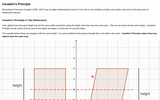Unrestricted Use
CC BY
Rating
0.0 stars
Overview:

Definition of Cavalieri’s Principle as it applies to two and three dimensional objects, with image examples.

Subject:
Geometry
Material Type:
Interactive
Provider:
Michigan Virtual
07/19/2019Rating
5.0 stars
Overview:

Students compare the relationships between radius, diameter, circumference, and area by manipulating a circle and increasing or decreasing its size in this interactive from Illuminations. It also provides a problems section where students determine the answer to each question using the applet, a calculator, or paper and pencil. They must also indicate the proper units!

Subject:
Mathematics
Geometry
Material Type:
Activity/Lab
Interactive
Provider:
National Council of Teachers of Mathematics
Author:
Illuminations
02/26/2019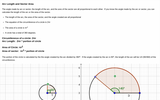Unrestricted Use
CC BY
Rating
3.0 stars
Overview:

Proportionality between circumference, angle and arc length; area, angle and area of sector. Circumference and area of circle equation, Image of four circles with hotpots of calculations

Subject:
Geometry
Material Type:
Interactive
Provider:
Michigan Virtual
07/19/2019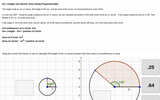Unrestricted Use
CC BY
Rating
2.0 stars
Overview:

Angle of sector or arc is proportional to the circumference or area of total circle. Interactive drag and drop factor to use when calculating arc length or sector area.

Subject:
Geometry
Material Type:
Interactive
Provider:
Michigan Virtual
07/19/2019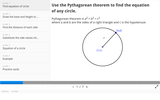Unrestricted Use
CC BY
Rating
2.0 stars
Overview:

Slides showing steps to derive the equation of a circle, using the Pythagorean Theorem

Subject:
Geometry
Material Type:
Interactive
Provider:
Michigan Virtual
07/19/2019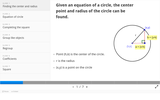Unrestricted Use
CC BY
Rating
3.0 stars
Overview:

Slides showing steps to solve for center and radius of a circle given an equation of a circle by completing the square.

Subject:
Geometry
Material Type:
Interactive
Provider:
Michigan Virtual
07/19/2019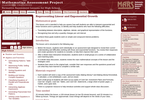Only Sharing Permitted
CC BY-NC-ND
Rating
4.0 stars
Overview:

This lesson unit is intended to help teachers assess how well students are able to interpret exponential and linear functions and in particular to identify and help students who have the following difficulties: translating between descriptive, algebraic and tabular data, and graphical representation of the functions; recognizing how, and why, a quantity changes per unit intervale; and to achieve these goals students work on simple and compound interest problems.

Subject:
Algebra
Geometry
Material Type:
Assessment
Lesson Plan
Provider:
Shell Center for Mathematical Education
Provider Set:
Mathematics Assessment Project (MAP)
06/24/2019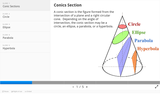Unrestricted Use
CC BY
Rating
2.33333333333 stars
Overview:

Image showing four conic sections, with an example of each type of equation; circle, ellipse, parabola, and hyperbola.

Subject:
Geometry
Material Type:
Interactive
Provider:
Michigan Virtual
07/19/2019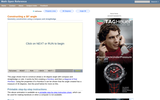Rating
0.0 stars
Overview:

An interactive applet and associated web page that show how to construct a 30 degrees angle with a compass and straightedge. The animation can be single-stepped or run as a continuous movie. Applet can be enlarged to full screen size for use with a classroom projector. This resource is a component of the Math Open Reference Interactive Geometry textbook project at http://www.mathopenref.com.

Subject:
Geometry
Material Type:
Simulation
Provider:
Math Open Reference
Author:
John Page
05/07/2019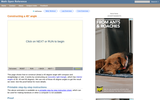Rating
0.0 stars
Overview:

An interactive applet and associated web page that show how to construct a 45 degrees angle with a compass and straightedge. The animation can be single-stepped or run as a continuous movie. Applet can be enlarged to full screen size for use with a classroom projector. This resource is a component of the Math Open Reference Interactive Geometry textbook project at http://www.mathopenref.com.

Subject:
Geometry
Material Type:
Simulation
Provider:
Math Open Reference
Author:
John Page
05/07/2019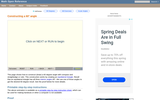Rating
0.0 stars
Overview:

An interactive applet and associated web page that show how to construct a 60 degrees angle with a compass and straightedge. The animation can be single-stepped or run as a continuous movie. Applet can be enlarged to full screen size for use with a classroom projector. This resource is a component of the Math Open Reference Interactive Geometry textbook project at http://www.mathopenref.com.

Subject:
Geometry
Material Type:
Simulation
Provider:
Math Open Reference
Author:
John Page
05/07/2019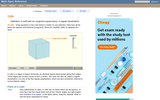Rating
3.0 stars
Overview:

An interactive applet and associated web page that demonstrate the properties of a cube. A 3-D cube is shown in the applet which can be interactively manipulated using the mouse. Research has shown that some younger students have difficulty visualizing the parts of a 3D object that are hidden. To help with this, the student can rotate the cube in any axis simply by dragging it with the mouse. It can also be 'exploded' - where a slider gradually separates the faces to reveal the ones behind. The cube can also be made translucent so you see through it to the other side. Applet can be enlarged to full screen size for use with a classroom projector, and printed to make handouts. This resource is a component of the Math Open Reference Interactive Geometry textbook project at http://www.mathopenref.com.

Subject:
Geometry
Material Type:
Simulation
Provider:
Math Open Reference
Author:
John Page
05/07/2019Rating
0.0 stars
Overview:

Students explore the volume of a box based on the amount of unit cubes that can fit inside of it in this student interactive, from Illuminations. They are prompted to come up with a rule for determining the volume of a box when its width, depth, and height are known.

Subject:
Mathematics
Geometry
Material Type:
Activity/Lab
Interactive
Simulation
Provider:
National Council of Teachers of Mathematics
Author:
Illuminations
02/26/2019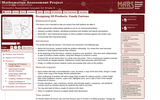Only Sharing Permitted
CC BY-NC-ND
Rating
3.0 stars
Overview:

This lesson unit is intended to help teachers assess how well students are able to: Select appropriate mathematical methods to use for an unstructured problem; interpret a problem situation, identifying constraints and variables, and specify assumptions; work with 2- and 3-dimensional shapes to solve a problem involving capacity and surface area; and communicate their reasoning clearly.

Subject:
Geometry
Material Type:
Assessment
Lesson Plan
Provider:
Shell Center for Mathematical Education
Provider Set:
Mathematics Assessment Project (MAP)
07/31/2019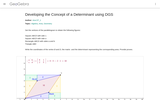Conditional Remix & Share Permitted
CC BY-SA
Rating
0.0 stars
Overview:

Developing the Concept of a Determinant using DGS

Subject:
Algebra
Geometry
Material Type:
Simulation
Provider:
GeoGebra
Provider Set:
GeoGebraTube
11/11/2011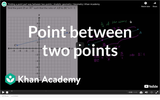Unrestricted Use
CC BY
Rating
4.0 stars
Overview:

Khan Academy video describing using coordinate plane to divide a line segment into a 3:1 ratio. Interactive drag and drop for estimating point, and button for additional explanation regarding ratio is added.

Subject:
Geometry
Material Type:
Interactive
Provider:
Michigan Virtual
07/19/2019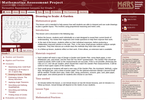Only Sharing Permitted
CC BY-NC-ND
Rating
3.5 stars
Overview:

This lesson unit is intended to help assess how well students are able to interpret and use scale drawings to plan a garden layout. This involves using proportional reasoning and metric units.

Subject:
Algebra
Geometry
Material Type:
Assessment
Lesson Plan
Provider:
Shell Center for Mathematical Education
Provider Set:
Mathematics Assessment Project (MAP)
06/24/2019Rating
0.0 stars
Overview:

Create customized activity sheets for your classroom! Nets of 3-D shapes, tessellations, coordinate graphs, and more - all based on your input.

Subject:
Mathematics
Geometry
Material Type:
Activity/Lab
Interactive
Simulation
Provider:
National Council of Teachers of Mathematics
Author:
Illuminations
02/26/2019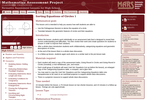Only Sharing Permitted
CC BY-NC-ND
Rating
4.5 stars
Overview:

This lesson unit is intended to help teachers assess how well students are able to: use the Pythagorean theorem to derive the equation of a circle; and translate between the geometric features of circles and their equations.

Subject:
Geometry
Material Type:
Assessment
Lesson Plan
Provider:
Shell Center for Mathematical Education
Provider Set:
Mathematics Assessment Project (MAP)
07/31/2019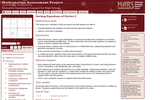Only Sharing Permitted
CC BY-NC-ND
Rating
4.0 stars
Overview:

This lesson unit is intended to help teachers assess how well students are able to: translate between the equations of circles and their geometric features; and sketch a circle from its equation.

Subject:
Geometry
Material Type:
Assessment
Lesson Plan
Provider:
Shell Center for Mathematical Education
Provider Set:
Mathematics Assessment Project (MAP)
07/31/2019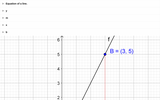Unrestricted Use
CC BY
Rating
2.0 stars
Overview:

Equation of a line with image, equations of parallel lines with image, equations of perpendicular lines with image.

Subject:
Geometry
Material Type:
Interactive
Provider:
Michigan Virtual
07/19/2019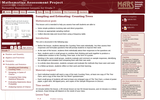Only Sharing Permitted
CC BY-NC-ND
Rating
4.0 stars
Overview:

This lesson unit is intended to help you assess how well students are able to: solve simple problems involving ratio and direct proportion; choose an appropriate sampling method; and collect discrete data and record them using a frequency table.

Subject:
Geometry
Material Type:
Assessment
Lecture Notes
Lesson Plan
Teaching/Learning Strategy
Provider:
Shell Center for Mathematical Education
Provider Set:
Mathematics Assessment Project (MAP)
Author:
http://map.mathshell.org/
06/24/2019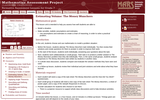Only Sharing Permitted
CC BY-NC-ND
Rating
4.0 stars
Overview:

This lesson unit is intended to help you assess how well students are able to: Model a situation; make sensible, realistic assumptions and estimates; and use assumptions and estimates to create a chain of reasoning, in order to solve a practical problem.

Subject:
Geometry
Material Type:
Assessment
Lesson Plan
Provider:
Shell Center for Mathematical Education
Provider Set:
Mathematics Assessment Project (MAP)
06/24/2019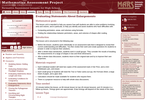Only Sharing Permitted
CC BY-NC-ND
Rating
4.0 stars
Overview:

This lesson unit is intended to help teachers assess how well students are able to solve problems involving area and volume, and in particular, to help you identify and assist students who have difficulties with the following: computing perimeters, areas and volumes using formulas; and finding the relationships between perimeters, areas, and volumes of shapes after scaling.

Subject:
Geometry
Material Type:
Assessment
Lesson Plan
Provider:
Shell Center for Mathematical Education
Provider Set:
Mathematics Assessment Project (MAP)
07/31/2019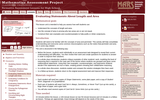Only Sharing Permitted
CC BY-NC-ND
Rating
4.0 stars
Overview:

This lesson unit is intended to help teachers assess how well students can: Understand the concepts of length and area; use the concept of area in proving why two areas are or are not equal; and construct their own examples and counterexamples to help justify or refute conjectures.

Subject:
Geometry
Material Type:
Assessment
Lesson Plan
Provider:
Shell Center for Mathematical Education
Provider Set:
Mathematics Assessment Project (MAP)
07/31/2019Conditional Remix & Share Permitted
CC BY-SA
Rating
4.0 stars
Overview:

Based on knowledge of the unit Circles, students see how sinusoids (Sine and coSine waves) get their shapes.

Subject:
Geometry
Material Type:
Simulation
Provider:
GeoGebra
Provider Set:
GeoGebraTube
04/26/2012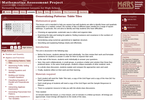Only Sharing Permitted
CC BY-NC-ND
Rating
5.0 stars
Overview:

This lesson unit is intended to help teachers assess how well students are able to identify linear and quadratic relationships in a realistic context: the number of tiles of different types that are needed for a range of square tabletops. In particular, this unit aims to identify and help students who have difficulties with: choosing an appropriate, systematic way to collect and organize data; examining the data and looking for patterns; finding invariance and covariance in the numbers of different types of tile; generalizing using numerical, geometrical or algebraic structure; and describing and explaining findings clearly and effectively.

Subject:
Algebra
Geometry
Material Type:
Assessment
Lesson Plan
Provider:
Shell Center for Mathematical Education
Provider Set:
Mathematics Assessment Project (MAP)
07/31/2019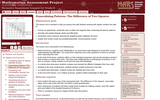Only Sharing Permitted
CC BY-NC-ND
Rating
5.0 stars
Overview:

This lesson unit is intended to help you assess how well students working with square numbers are able to: choose an appropriate, systematic way to collect and organize data, examining the data for patterns; describe and explain findings clearly and effectively; generalize using numerical, geometrical, graphical and/or algebraic structure; and explain why certain results are possible/impossible, moving towards a proof.

Subject:
Algebra
Geometry
Material Type:
Assessment
Lesson Plan
Provider:
Shell Center for Mathematical Education
Provider Set:
Mathematics Assessment Project (MAP)
07/31/2019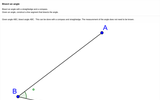Unrestricted Use
CC BY
Rating
2.0 stars
Overview:

Images of construction of angle bisector, with descriptive text and hotspots of key points on images.

Subject:
Geometry
Material Type:
Interactive
Provider:
Michigan Virtual
07/19/2019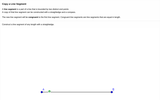Unrestricted Use
CC BY
Rating
2.0 stars
Overview:

Images of construction of copying a line segment, with descriptive text and hotspots of key points on images.

Subject:
Geometry
Material Type:
Interactive
Provider:
Michigan Virtual
07/19/2019Unrestricted Use
CC BY
Rating
2.0 stars
Overview:

Images of construction of copying a line segment, with descriptive text and hotspots of key points on images.

Subject:
Geometry
Material Type:
Interactive
Provider:
Michigan Virtual
07/19/2019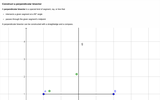Unrestricted Use
CC BY
Rating
2.0 stars
Overview:

Images of construction of perpendicular bisector, with descriptive text and hotspots of key points on images.

Subject:
Geometry
Material Type:
Interactive
Provider:
Michigan Virtual
07/19/2019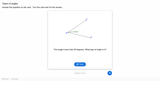Unrestricted Use
CC BY
Rating
4.0 stars
Overview:

11 dialog cards with images, each card contains an angle type, description, and definition of different types of angles.

Subject:
Geometry
Material Type:
Interactive
Provider:
Michigan Virtual
07/19/2019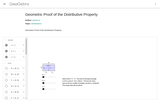Conditional Remix & Share Permitted
CC BY-NC-SA
Rating
0.0 stars
Overview:

This resource demonstrates the distributive property through the area of rectangles. You can manipulate the dimension by moving the slider.

Subject:
Mathematics
Algebra
Geometry
Material Type:
Diagram/Illustration
Simulation
Provider:
GeoGebra
Author:
jtmace11
07/31/2019Rating
0.0 stars
Overview:

Students to explore various geometric solids and their properties in this student interactive, from Illuminations. Students can virtually manipulate and color each solid to investigate properties such as the number of faces, edges, and vertices. Students can explore tetrahedrons, cubes, octahedrons, dodecahedrons, icosahedrons, and irregular polyhedrons by toggling between solid and net to see different views of the selected shape.

Subject:
Mathematics
Geometry
Material Type:
Activity/Lab
Interactive
Provider:
National Council of Teachers of Mathematics
Author:
Illuminations
02/26/2019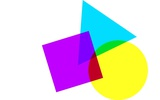Conditional Remix & Share Permitted
CC BY-NC-SA
Rating
0.0 stars
Overview:

This math unit teaches students to classify quadrilaterals into categories based on their properties; classify quadrilaterals in a hierarchy based on properties. This unit helps to explain that attributes belonging to a category of quadrilaterals also belong to all subcategories of that category.

Subject:
Discrete Math
Geometry
Material Type:
Lesson
Unit of Study
Author:
OMAR LEMUS
02/26/2022Unrestricted Use
CC BY
Rating
3.0 stars
Overview:

Dialog cards with image and definition of point, line, line segment, line, distance, angle, circle, parallel lines, perpendicular lines

Subject:
Geometry
Material Type:
Interactive
Provider:
Michigan Virtual
07/19/2019Unrestricted Use
CC BY
Rating
0.0 stars
Overview:

In this unit, children learn about attributes of shapes, compose and decompose composite shapes.

Subject:
Mathematics
Geometry
Material Type:
Unit of Study
Provider:
Michigan Virtual
Author:
Jen Duman
08/23/2016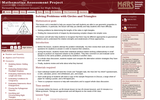Only Sharing Permitted
CC BY-NC-ND
Rating
5.0 stars
Overview:

This lesson unit is intended to help teachers assess how well students are able to use geometric properties to solve problems. In particular, the lesson will help you identify and help students who have the following difficulties: solving problems by determining the lengths of the sides in right triangles; and finding the measurements of shapes by decomposing complex shapes into simpler ones. The lesson unit will also help students to recognize that there may be different approaches to geometrical problems, and to understand the relative strengths and weaknesses of those approaches.

Subject:
Geometry
Material Type:
Assessment
Lesson Plan
Provider:
Shell Center for Mathematical Education
Provider Set:
Mathematics Assessment Project (MAP)